Courses

# Dynamic Programming, P & NP Concepts (Basic Level)

## 10 Questions MCQ Test Question Bank for GATE Computer Science Engineering | Dynamic Programming, P & NP Concepts (Basic Level)

Description
This mock test of Dynamic Programming, P & NP Concepts (Basic Level) for Computer Science Engineering (CSE) helps you for every Computer Science Engineering (CSE) entrance exam. This contains 10 Multiple Choice Questions for Computer Science Engineering (CSE) Dynamic Programming, P & NP Concepts (Basic Level) (mcq) to study with solutions a complete question bank. The solved questions answers in this Dynamic Programming, P & NP Concepts (Basic Level) quiz give you a good mix of easy questions and tough questions. Computer Science Engineering (CSE) students definitely take this Dynamic Programming, P & NP Concepts (Basic Level) exercise for a better result in the exam. You can find other Dynamic Programming, P & NP Concepts (Basic Level) extra questions, long questions & short questions for Computer Science Engineering (CSE) on EduRev as well by searching above.
QUESTION: 1

### A binary search tree is generated by inserting in order the following integers: 50, 15, 62, 5, 20, 58, 91,3, 8, 37,60, 24. The number of nodes in the left subtree and right subtree of the root respectively is

Solution:

The binary search tree is as follows: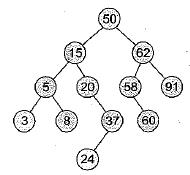The number of nodes in the left subtree and right subtree of the root respectively is (7, 4).

QUESTION: 2

### Which one of the following algorithm design techniques is used in finding all pairs of shortest distances in a graph?

Solution:

Bellman-Ford algorithm is used to find all pairs shortest distances in a graph and it is dynamic programming technique.

QUESTION: 3

### A B-tree of order 4 is built from scratch by 10 successive insertions. What is the maximum number of node splitting operations that may take place?

Solution:

A B-tree is similar to 2-3 tree. Consider a B-tree of order 4.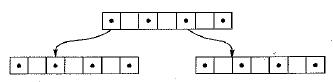A B-tree of order m contains n records and if each contains b records on the average then the tree has about [ n / b ] leaves, if we split k nodes along the path from leaves then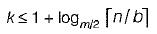in given problem n = 10, b = 3, m = 4

so,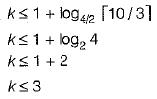QUESTION: 4

A problem in NP is NP-complete if

Solution:

3-SAT being an NPC problem, reducing NP problem to 3-SAT would mean that NP problem is NPC

QUESTION: 5

For problems X and Y, Y is NP-complete and X reduces to Y in polynomial time. Which of the following is TRUE?

Solution:

X is reducible to NPC.
Hence X is also NPC

QUESTION: 6

Let π be a problem that belongs to the class NP. Then which one of the following is TRUE?

Solution:

it is given that πA ∈ NP
∴ If πA is NP-hard, and since it is given that πA ∈ NP , this means that πA is NP-complete
∴ choice (c) is correct.
Notice that choice (a) is incorrect since as P ∈ NP, some NP problems are actually P and hence polynomial time algorithm can exist for these.
Choice (b) is incorrect since, If πA can be solved deterministicaily in polynomial time, it does not generate that P=NP, unless of-course it is additionally known that πA is NP-complete.
Choice (d) is incorrect since,
All problems belonging to P or NP have to be decidable.

QUESTION: 7

The maximum number of edges in a n-nodes undirected graph without self loops is

Solution:

The maximum number of edges in a n-node undirected graph without self loop i.e., complete graph.
n-node each having degree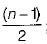such each  edge so total number of edges =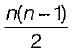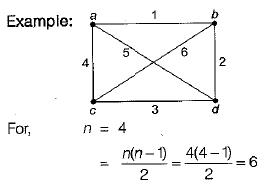QUESTION: 8

The average number of key comparisons required for a successful search for sequential search on n items is

Solution:

Number of comparison in worst case = n

Number of comparison in best case = 1 So, average number of comparison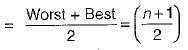QUESTION: 9

A complete binary tree with n non-leaf nodes contains

Solution:

In complete binary tree.
Number of internal nodes = No. of leaf node - 1
So total number of nodes = Internal nodes + Leaf node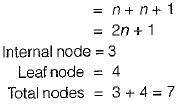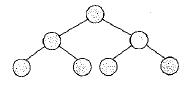QUESTION: 10

Algorithm design technique used in quicksort algorithm is

Solution:

Quick sort algorithm is based on divide an conquer approach.
Since we conquer the array by dividing it one the basis of pivot elements till the sorted array is obtained